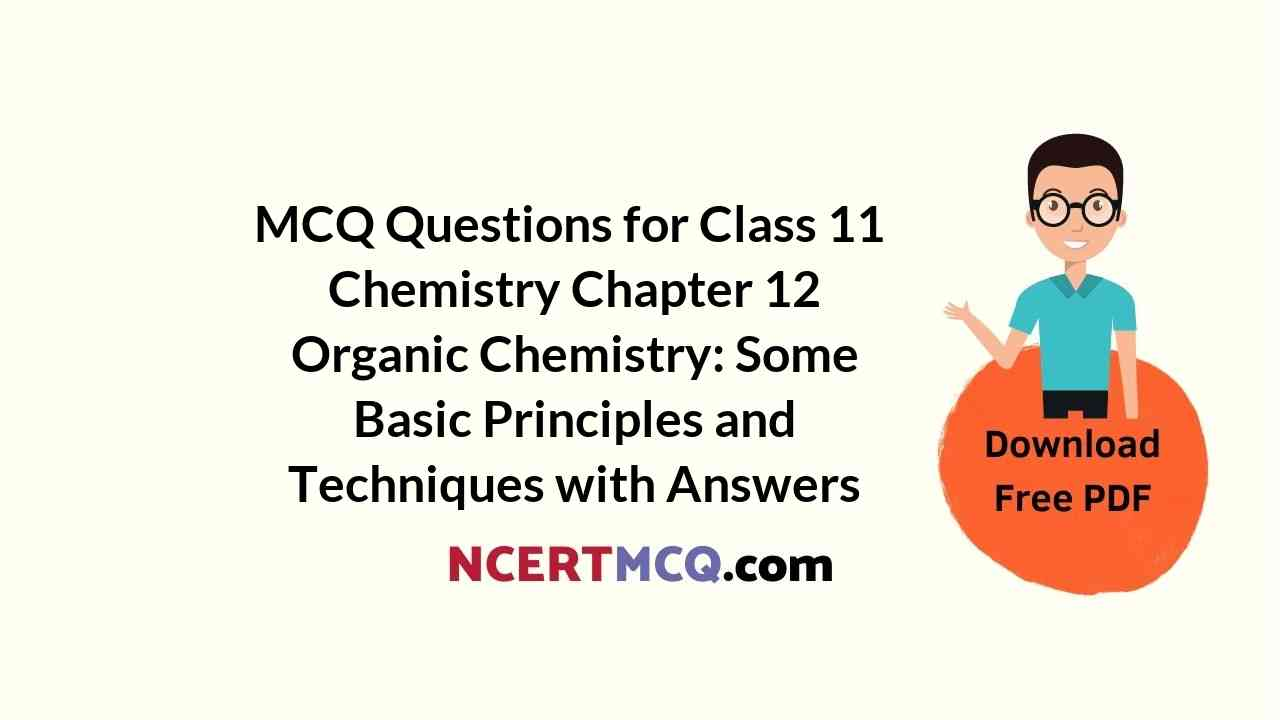Check the below NCERT MCQ Questions for Class 11 Chemistry Chapter 12 Organic Chemistry: Some Basic Principles and Techniques with Answers Pdf free download. MCQ Questions for Class 11 Chemistry with Answers were prepared based on the latest exam pattern. We have provided Organic Chemistry: Some Basic Principles and Techniques Class 11 Chemistry MCQs Questions with Answers to help students understand the concept very well.

## Organic Chemistry: Some Basic Principles and Techniques Class 11 MCQs Questions with Answers

Organic Chemistry Class 11 MCQ Question 1.
If two compounds have the same empirical formula but different molecular formula they must have
(a) Different percentage composition
(b) Different molecular weight
(c) Same viscosity
(d) Same vapour density

MCQ On Organic Chemistry Class 11 Question 2.
Identify the chiral molecule among the following:
(a) Isopropyl alcohol
(b) 2-pentanol
(c) 1-bromo 3-butene
(d) Isobutyl alcohol

Explanation:
Chirality is the condition for a molecule to be optically active and here isobutyl alcohol is the only compound is optically active and hence it is the chiral molecule.

Class 11 Organic Chemistry MCQ Question 3.
0.0833mol of carbohydrate of empirical formula CH2O contain 1g of hydrogen. The molecular formula of the carbohydrate is
(a) C5H10O5
(b) C3H4O3
(c) C12H22O11
(d) C6H12O6

Explanation:
As 0.0833 mole carbohydrate has hydrogen = 1g
Therefore, 1 mole carbohydrate has hydrogen = ($$\frac {1}{0.0833}$$) = 12g
Empirical Formula (CH2O) has hydrogen = 2g
Hence n = $$\frac {(12)}{(2)}$$ = 6
Hence molecular formula of carbohydrate =(CH2O)6 = C6H12O6

MCQ Of Organic Chemistry Class 11 Question 4.
The displacement of electrons in a multiple bond in the presence of attacking reagent is called
(a) Inductive effect
(b) Electromeric effect
(c) Resonance
(d) Hyper conjugation.

Explanation:
The electromeric effect is a temporary effect brought into play at the requirement of attacking reagent. Electromeric effect refers to a molecular polarizability effect occurring by an intra-molecular electron displacement. It is the temporary effect.

Class 11 Chemistry Chapter 12 MCQ Question 5.
Which of the following cannot be represented by resonance structures?
(a) Dimethyl ether
(b) Nitrate anion
(c) Carboxylate anion
(d) Toluene

Explanation:
Ethers due to absence of delocalized pair of electrons do not show resonance.

Organic Chemistry MCQ Class 11 Question 6.
An organic compound which produces a bluish green coloured flame on heating in presence of copper is
(a) Chlorobenzene
(b) Benzaldehyde
(c) Aniline
(d) Benzoic acid

Explanation:
Halogen containing compounds, e.g.,C6H5Cl when placed in a flame, the presence of halogen is revealed by a green to blue flame.

Some Basic Concepts Of Organic Chemistry Class 11 MCQ With Answers Question 7.
Which one is strongest acid among following options?
(a) CH2FCOOH
(b) CH2ClCOOH
(c) CHCl2COOH
(d) CHF2COOH

Explanation:
CHF2−COOH. Difluoroacetic acid is strongest because presence of two F atoms increases its acidic nature.

Organic Chemistry Class 11 MCQ Pdf Question 8.
Insulin contains 3.4% sulphur. The minimum molecular weight of insulin is
(a) 350
(b) 470
(c) 560
(d) 940

Explanation:
Minimum mass of sulphur = wt. of its one atom = 32
As 3.4 gms of sulphur present in 100 gms.
Therefore, 32 gms of sulphur present in = (100 × 32)/(3.4) = 940

Organic Chemistry Class 11 MCQs Question 9.
What is the correct IUPAC name of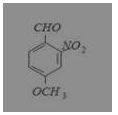(a) 4-methoxy-2-nitrobenzaldehyde
(b) 4-formyl-3-nitro anisole
(c) 4-methoxy-6-nitrobenzaldehyde
(d) 2-formyl-5-methoxy nitrobenzene

Explanation:
IUPAC name is 4-methoxy-2-nitrobenzaldehyde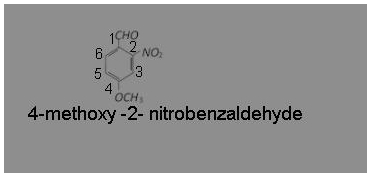Organic Chemistry Some Basic Principles And Techniques MCQ Question 10.
59 g of an amide obtained from a carboxylic acid, RCOOH, liberated 17 g of ammonia upon heating with alkali. The acid is
(a) Formic Acid
(b) Acetic Acid
(c) Propionic Acid
(d) Benzoic Acid

Explanation:
RCOOH → RCONH2 → NH3
Since, 17g of NH3 is liberated from 59 g of acid amide, the amide has molecular mass of 59, i.e., RCONH2 = 59
R + 12 + 16 + 14 + 2 = 59
R + 44 = 59
R = 15
Hence, RisCH3 group and thus acid is CH3COOH(Acetic acid)

MCQ Questions For Class 11 Chemistry Chapter 12 Question 11.
In the Dumas method, the nitrogen present in organic compound gets converted to
(a) Sodium Cyanide
(b) Gaseoue Ammonia
(c) Dinitrogen Gas
(d) Ammonium Sulphate.

Explanation:
Nitrogen present in the organic compound is converted into N2 gas by heating the compound with CuO.

Ch 12 Chemistry Class 11 MCQ Question 12.
0.0833mol of carbohydrate of empirical formula CH2O contain 1 g of hydrogen. The molecular formula of the carbohydrate is
(a) C5H10O5
(b) C3H4O3
(c) C12H22O11
(d) C6H12O6

Explanation:
As 0.0833 mole carbohydrate has hydrogen = 1 g
Therefore, 1 mole carbohydrate has hydrogen = ($$\frac {1}{0.0833}$$) = 12 g
Empirical Formula (CH2O) has hydrogen = 2 g
Hence n = $$\frac {(12)}{(2)}$$ = 6
Hence molecular formula of carbohydrate = (CH2O)6 = C6H12O6

Class 11 Chemistry Ch 12 MCQ Question 13.
The compound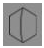is known by which of the following names
(a) Bicyclo-[2, 2, 2] octane
(b) Bicyclo-[2, 2, 1] octane
(c) Bicyclo-[1, 2, 1] octane
(d) Bicyclo-[1, 1, 1] octane

Answer: (a) Bicyclo-[2, 2, 2] octane
Explanation:
Bicyclo-[2, 2, 2] octane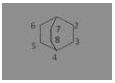MCQ Of Chapter Organic Chemistry Class 11 Question 14.
Which one of the following conformations of cyclohexane is chiral?
(a) Twist boat
(b) Rigid
(c) Chair
(d) Boat

Explanation:
The twist boat conformation of cyclohexane is optically active as it does not have any plane of symmetry.Class 11 Chemistry Chapter 12 MCQ With Answers Question 15.
If 0.228 g of silver salt of dibasic acid gave a residue of 0.162 g of silver on ignition then molecular weight of the acid is
(a) 70
(b) 80
(c) 90
(d) 100

Explanation:
Mass of silver salt taken = 0.228 gm
Mass of silver left = 0.162 gm
Basicity of acid = 2
Step 1- To calculate the equivalent mass of the silver salt (Eq. mass of silver salt)/(Eq. mass of silver)=(Mass of Acid taken)/(Mass of silver left)
($$\frac {E}{108}$$) = ($$\frac {0.228}{0.162}$$)
E = ($$\frac {0.228}{0.162}$$) × 108 = 152(Eq. mass of silver salt)
Step 2 – To calculate the eq. mass of acid = ( Equivalent mass of acid)
= Equivalent mass of silver salt – Equivalent mass of Ag + Basicity
= 152 – 108 + 1
= 152 – 109
= 43 (Equivalent mass of acid)
Step 3- To determine the molecular mass of acid. molecular mass of the acid = Equivalent mass of acid × basicity = 45 × 2 = 90.

Question 16.
If there is no rotation of plane polarized light by a compound in a specific solvent, thought to be chiral, it may mean that
(a) The compound may be a racemic mixture
(b) The compound is certainly a chiral
(c) The compound is certainly meso
(d) There is no compound in the solvent.

Answer: (c) The compound is certainly meso
Explanation:
Meso compound does not rotate plane polarised light. Compound which contains tetrahedral atoms with four different groups but the whole molecule is a chiral, is known as meso compound. It possesses a plane of symmetry and is optically inactive. One of the asymmetric carbon atoms turns the plane of polarised light to the right and other to the left and to the same extent so that the rotation due to upper half is compensated by the lower half, i.e., internally compensated, and finally there is no rotation of plane polarised light.

Question 17.
Which element is estimated by Carius method
(a) Carbon
(b) Hydrogen
(c) Halogen
(d) Nitrogen

Explanation:
Halogen element is estimated by Carius method

Question 18.
Inductive effect involves
(a) displacement of σ electrons
(b) delocalization of π electrons
(c) delocalization of σ-electrons
(d) displacement of π-electrons

Answer: (a) displacement of σ electrons
Explanation:
During inductive effect shifting of a electrons takes place due to which partially charges are developed on the atom.
+δ” +δ′ +δ −δ
C− C− C −Cl−

Question 19.
A crystalline solid possess which one of the following property?
(a) Irregularity
(b) Non- symmetric
(c) Perfect geometric pattern
(d) non- stability

Explanation:
A crystalline solid is one which possesses perfect geometry, high stability, symmetric and regularly arranged.

Question 20.
Which of the following behaves both as a nucleophile and as an electrophile?
(a) CH3C ≡ N
(b) CH3OH
(c) CH2 = CHCH3
(d) CH3NH2